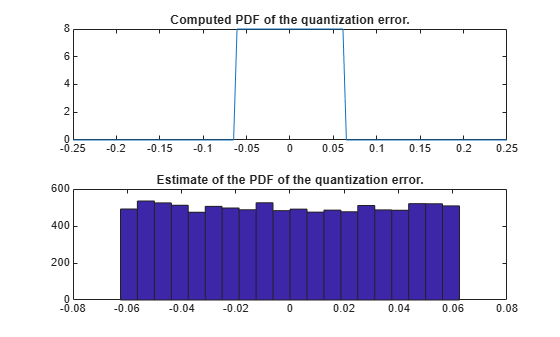# errpdf

Probability density function of quantization error

## Syntax

```[f,x] = errpdf(q) f = errpdf(q,x) ```

## Description

`[f,x] = errpdf(q)` returns the probability density function `f` evaluated at the values in `x`. The vector `x` contains the uniformly distributed random quantization errors that arise from quantizing a signal by `quantizer` object `q`.

`f = errpdf(q,x)` returns the probability density function `f` evaluated at the values in vector `x`.

Note

The results are not exact when the signal precision is close to the precision of the `quantizer`.

## Examples

collapse all

```q = quantizer('nearest',[4 3]); [f,x] = errpdf(q); subplot(211) plot(x,f) title('Computed PDF of the quantization error.')```The output plot shows the probability density function of the quantization error. Compare this result to a plot of the sample probability density function from a Monte Carlo experiment:

``` r = realmax(q); u = 2*r*rand(10000,1)-r; % Original signal y = quantize(q,u); % Quantized signal e = y - u; % Error subplot(212) hist(e,20) gca.xlim = [min(x) max(x)]; title('Estimate of the PDF of the quantization error.')```